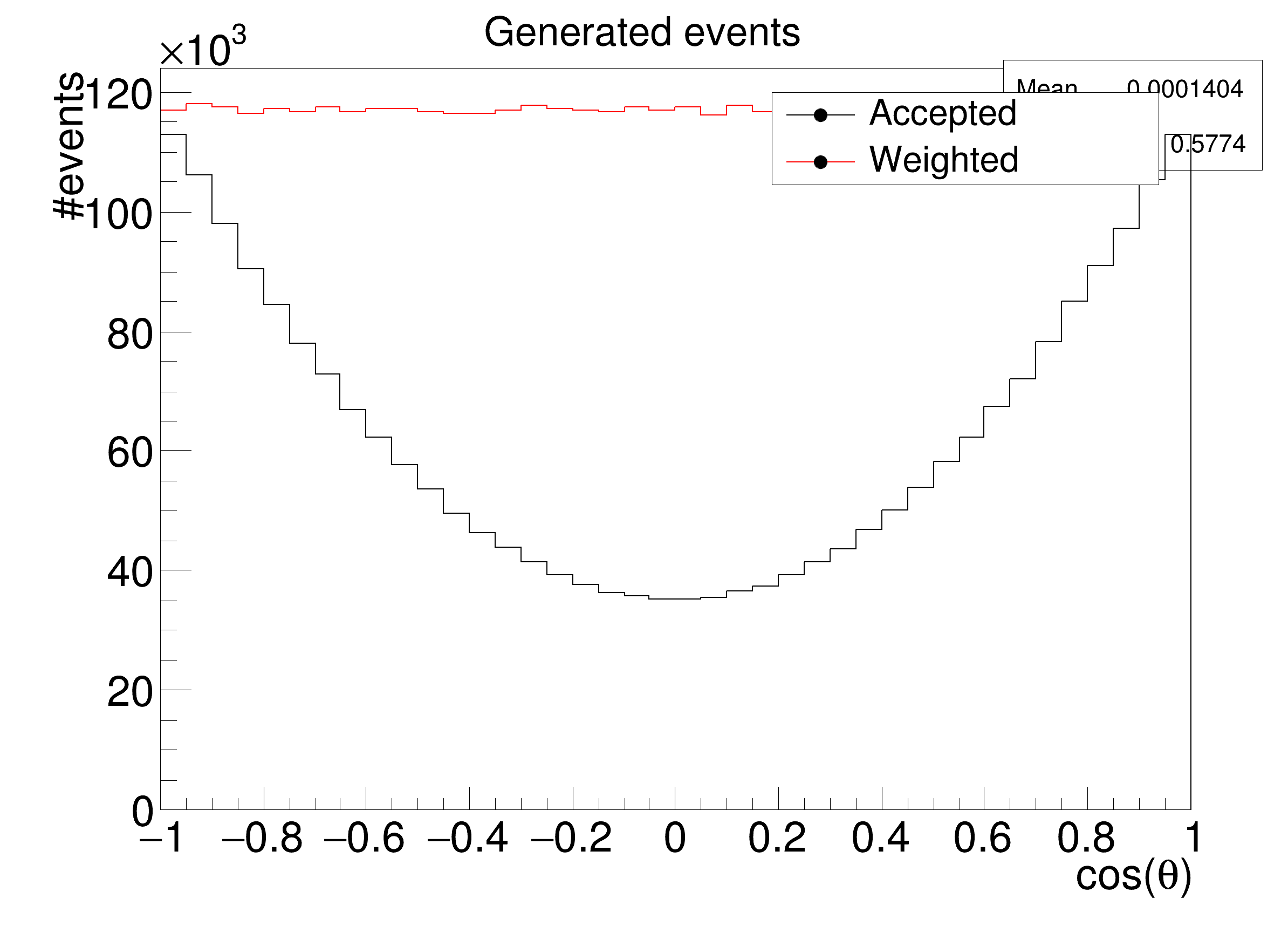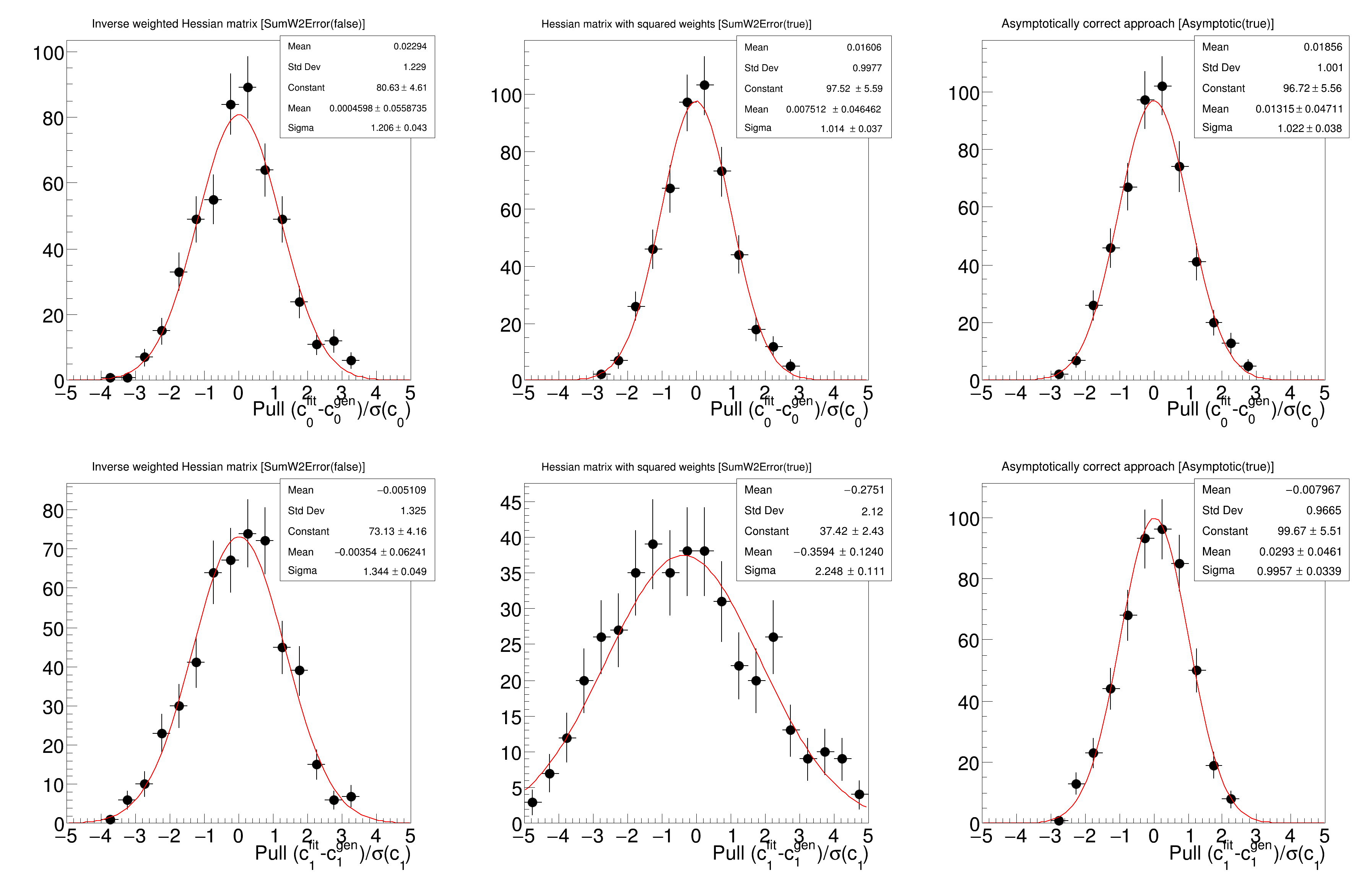ROOT   Reference Guiderf611_weightedfits.C File Reference

## Detailed Description

Likelihood and minimization: Parameter uncertainties for weighted unbinned ML fits

## Parameter uncertainties for weighted unbinned ML fits

Based on example from https://arxiv.org/abs/1911.01303

This example compares different approaches to determining parameter uncertainties in weighted unbinned maximum likelihood fits. Performing a weighted unbinned maximum likelihood fits can be useful to account for acceptance effects and to statistically subtract background events using the sPlot formalism. It is however well known that the inverse Hessian matrix does not yield parameter uncertainties with correct coverage in the presence of event weights. Three approaches to the determination of parameter uncertainties are compared in this example:

1. Using the inverse weighted Hessian matrix [SumW2Error(false)]
2. Using the expression [SumW2Error(true)]

$V_{ij} = H_{ik}^{-1} C_{kl} H_{lj}^{-1}$

where H is the weighted Hessian matrix and C is the Hessian matrix with squared weights
3. The asymptotically correct approach (for details please see https://arxiv.org/abs/1911.01303) [Asymptotic(true)]

$V_{ij} = H_{ik}^{-1} D_{kl} H_{lj}^{-1}$

where H is the weighted Hessian matrix and D is given by

$D_{kl} = \sum_{e=1}^{N} w_e^2 \frac{\partial \log(P)}{\partial \lambda_k}\frac{\partial \log(P)}{\partial \lambda_l}$

with the event weight $$w_e$$.

The example performs the fit of a second order polynomial in the angle cos(theta) [-1,1] to a weighted data set. The polynomial is given by

$P = \frac{ 1 + c_0 \cdot \cos(\theta) + c_1 \cdot \cos(\theta) \cdot \cos(\theta) }{\mathrm{Norm}}$

The two coefficients $$c_0$$ and $$c_1$$ and their uncertainties are to be determined in the fit.

The per-event weight is used to correct for an acceptance effect, two different acceptance models can be studied:

• acceptancemodel==1: eff = $$0.3 + 0.7 \cdot \cos(\theta) \cdot \cos(\theta)$$
• acceptancemodel==2: eff = $$1.0 - 0.7 \cdot \cos(\theta) \cdot \cos(\theta)$$ The data is generated to be flat before the acceptance effect.

The performance of the different approaches to determine parameter uncertainties is compared using the pull distributions from a large number of pseudoexperiments. The pull is defined as $$(\lambda_i - \lambda_{gen})/\sigma(\lambda_i)$$, where $$\lambda_i$$ is the fitted parameter and $$\sigma(\lambda_i)$$ its uncertainty for pseudoexperiment number i. If the fit is unbiased and the parameter uncertainties are estimated correctly, the pull distribution should be a Gaussian centered around zero with a width of one.[1mRooFit v3.60 -- Developed by Wouter Verkerke and David Kirkby[0m
Copyright (C) 2000-2013 NIKHEF, University of California & Stanford University
Running 1500 toy fits ...
... done.
FCN=13.515 FROM MIGRAD STATUS=CONVERGED 60 CALLS 61 TOTAL
EDM=7.11482e-10 STRATEGY= 1 ERROR MATRIX ACCURATE
EXT PARAMETER STEP FIRST
NO. NAME VALUE ERROR SIZE DERIVATIVE
1 Constant 8.06326e+01 4.61035e+00 6.80051e-03 2.63179e-06
2 Mean 4.59821e-04 5.58735e-02 1.02835e-04 -6.59629e-04
3 Sigma 1.20608e+00 4.29699e-02 1.71309e-05 1.24617e-03
FCN=5.84356 FROM MIGRAD STATUS=CONVERGED 59 CALLS 60 TOTAL
EDM=4.43987e-09 STRATEGY= 1 ERROR MATRIX ACCURATE
EXT PARAMETER STEP FIRST
NO. NAME VALUE ERROR SIZE DERIVATIVE
1 Constant 9.75213e+01 5.59338e+00 5.60402e-03 -1.97617e-05
2 Mean 7.51216e-03 4.64617e-02 5.90804e-05 -7.04982e-04
3 Sigma 1.01358e+00 3.70947e-02 1.21766e-05 -6.78934e-03
FCN=5.92321 FROM MIGRAD STATUS=CONVERGED 59 CALLS 60 TOTAL
EDM=2.82374e-09 STRATEGY= 1 ERROR MATRIX ACCURATE
EXT PARAMETER STEP FIRST
NO. NAME VALUE ERROR SIZE DERIVATIVE
1 Constant 9.67219e+01 5.56424e+00 5.59080e-03 -1.59410e-05
2 Mean 1.31546e-02 4.71082e-02 5.99842e-05 -5.99127e-04
3 Sigma 1.02204e+00 3.77681e-02 1.23082e-05 -4.76847e-03
FCN=9.99353 FROM MIGRAD STATUS=CONVERGED 51 CALLS 52 TOTAL
EDM=1.54209e-08 STRATEGY= 1 ERROR MATRIX ACCURATE
EXT PARAMETER STEP FIRST
NO. NAME VALUE ERROR SIZE DERIVATIVE
1 Constant 7.31330e+01 4.15612e+00 5.34878e-03 8.14697e-06
2 Mean -3.53982e-03 6.24075e-02 1.00776e-04 2.69686e-03
3 Sigma 1.34426e+00 4.86401e-02 1.55414e-05 6.26796e-03
FCN=13.9377 FROM MIGRAD STATUS=CONVERGED 59 CALLS 60 TOTAL
EDM=1.41305e-07 STRATEGY= 1 ERROR MATRIX ACCURATE
EXT PARAMETER STEP FIRST
NO. NAME VALUE ERROR SIZE DERIVATIVE
1 Constant 3.74207e+01 2.42528e+00 3.48790e-03 -5.82511e-05
2 Mean -3.59360e-01 1.24029e-01 2.28239e-04 3.37657e-03
3 Sigma 2.24823e+00 1.11069e-01 2.42149e-05 -2.95187e-02
FCN=6.06878 FROM MIGRAD STATUS=CONVERGED 60 CALLS 61 TOTAL
EDM=2.14707e-09 STRATEGY= 1 ERROR MATRIX ACCURATE
EXT PARAMETER STEP FIRST
NO. NAME VALUE ERROR SIZE DERIVATIVE
1 Constant 9.96656e+01 5.51125e+00 5.82217e-03 -7.19807e-06
2 Mean 2.92964e-02 4.61022e-02 5.98483e-05 1.20218e-03
3 Sigma 9.95652e-01 3.38750e-02 1.20391e-05 -3.38249e-03
(int) 0
#include "TH1D.h"
#include "TCanvas.h"
#include "TROOT.h"
#include "TStyle.h"
#include "TRandom3.h"
#include "TLegend.h"
#include "RooRealVar.h"
#include "RooFitResult.h"
#include "RooDataSet.h"
#include "RooPolynomial.h"
using namespace RooFit;
int rf611_weightedfits(int acceptancemodel=2) {
// I n i t i a l i s a t i o n a n d S e t u p
//------------------------------------------------
//plotting options
gStyle->SetTitleSize(0.05, "XY");
gStyle->SetLabelSize(0.05, "XY");
gStyle->SetTitleOffset(0.9, "XY");
//initialise TRandom3
TRandom3* rnd = new TRandom3();
rnd->SetSeed(191101303);
//accepted events and events weighted to account for the acceptance
TH1D* haccepted = new TH1D("haccepted", "Generated events;cos(#theta);#events", 40, -1.0, 1.0);
TH1D* hweighted = new TH1D("hweighted", "Generated events;cos(#theta);#events", 40, -1.0, 1.0);
//histograms holding pull distributions
//using the inverse Hessian matrix
TH1D* hc0pull1 = new TH1D("hc0pull1", "Inverse weighted Hessian matrix [SumW2Error(false)];Pull (c_{0}^{fit}-c_{0}^{gen})/#sigma(c_{0});", 20, -5.0, 5.0);
TH1D* hc1pull1 = new TH1D("hc1pull1", "Inverse weighted Hessian matrix [SumW2Error(false)];Pull (c_{1}^{fit}-c_{1}^{gen})/#sigma(c_{1});", 20, -5.0, 5.0);
//using the correction with the Hessian matrix with squared weights
TH1D* hc0pull2 = new TH1D("hc0pull2", "Hessian matrix with squared weights [SumW2Error(true)];Pull (c_{0}^{fit}-c_{0}^{gen})/#sigma(c_{0});", 20, -5.0, 5.0);
TH1D* hc1pull2 = new TH1D("hc1pull2", "Hessian matrix with squared weights [SumW2Error(true)];Pull (c_{1}^{fit}-c_{1}^{gen})/#sigma(c_{1});", 20, -5.0, 5.0);
//asymptotically correct approach
TH1D* hc0pull3 = new TH1D("hc0pull3", "Asymptotically correct approach [Asymptotic(true)];Pull (c_{0}^{fit}-c_{0}^{gen})/#sigma(c_{0});", 20, -5.0, 5.0);
TH1D* hc1pull3 = new TH1D("hc1pull3", "Asymptotically correct approach [Asymptotic(true)];Pull (c_{1}^{fit}-c_{1}^{gen})/#sigma(c_{1});", 20, -5.0, 5.0);
//number of pseudoexperiments (toys) and number of events per pseudoexperiment
constexpr unsigned int ntoys = 500;
constexpr unsigned int nstats = 5000;
//parameters used in the generation
constexpr double c0gen = 0.0;
constexpr double c1gen = 0.0;
// Silence fitting and minimisation messages
auto& msgSv = RooMsgService::instance();
msgSv.getStream(1).removeTopic(RooFit::Minimization);
msgSv.getStream(1).removeTopic(RooFit::Fitting);
std::cout << "Running " << ntoys*3 << " toy fits ..." << std::endl;
// M a i n l o o p : r u n p s e u d o e x p e r i m e n t s
//----------------------------------------------------------------
for (unsigned int i=0; i<ntoys; i++) {
//S e t u p p a r a m e t e r s a n d P D F
//-----------------------------------------------
//angle theta and the weight to account for the acceptance effect
RooRealVar costheta("costheta","costheta", -1.0, 1.0);
RooRealVar weight("weight","weight", 0.0, 1000.0);
//initialise parameters to fit
RooRealVar c0("c0","0th-order coefficient", c0gen, -1.0, 1.0);
RooRealVar c1("c1","1st-order coefficient", c1gen, -1.0, 1.0);
c0.setError(0.01);
c1.setError(0.01);
//create simple second-order polynomial as probability density function
RooPolynomial pol("pol", "pol", costheta, RooArgList(c0, c1), 1);
//G e n e r a t e d a t a s e t f o r p s e u d o e x p e r i m e n t i
//-------------------------------------------------------------------------------
RooDataSet data("data","data",RooArgSet(costheta, weight), WeightVar("weight"));
//generate nstats events
for (unsigned int j=0; j<nstats; j++) {
bool finished = false;
//use simple accept/reject for generation
while (!finished) {
costheta = 2.0*rnd->Rndm()-1.0;
//efficiency for the specific value of cos(theta)
double eff = 1.0;
if (acceptancemodel == 1)
eff = 1.0 - 0.7 * costheta.getVal()*costheta.getVal();
else
eff = 0.3 + 0.7 * costheta.getVal()*costheta.getVal();
//use 1/eff as weight to account for acceptance
weight = 1.0/eff;
//accept/reject
if (10.0*rnd->Rndm() < eff*pol.getVal())
finished = true;
}
haccepted->Fill(costheta.getVal());
hweighted->Fill(costheta.getVal(), weight.getVal());
}
//F i t t o y u s i n g t h e t h r e e d i f f e r e n t a p p r o a c h e s t o u n c e r t a i n t y d e t e r m i n a t i o n
//-------------------------------------------------------------------------------------------------------------------------------------------------
//this uses the inverse weighted Hessian matrix
RooFitResult* result = pol.fitTo(data, Save(true), SumW2Error(false), PrintLevel(-1), BatchMode(true));
hc0pull1->Fill((c0.getVal()-c0gen)/c0.getError());
hc1pull1->Fill((c1.getVal()-c1gen)/c1.getError());
//this uses the correction with the Hesse matrix with squared weights
result = pol.fitTo(data, Save(true), SumW2Error(true), PrintLevel(-1), BatchMode(true));
hc0pull2->Fill((c0.getVal()-c0gen)/c0.getError());
hc1pull2->Fill((c1.getVal()-c1gen)/c1.getError());
//this uses the asymptotically correct approach
result = pol.fitTo(data, Save(true), AsymptoticError(true), PrintLevel(-1), BatchMode(true));
hc0pull3->Fill((c0.getVal()-c0gen)/c0.getError());
hc1pull3->Fill((c1.getVal()-c1gen)/c1.getError());
}
std::cout << "... done." << std::endl;
// P l o t o u t p u t d i s t r i b u t i o n s
//--------------------------------------------------
//plot accepted (weighted) events
TCanvas* cevents = new TCanvas("cevents", "cevents", 800, 600);
cevents->cd(1);
hweighted->SetMinimum(0.0);
hweighted->SetLineColor(2);
hweighted->Draw("hist");
haccepted->Draw("same hist");
TLegend* leg = new TLegend(0.6, 0.8, 0.9, 0.9);
leg->Draw();
cevents->Update();
//plot pull distributions
TCanvas* cpull = new TCanvas("cpull", "cpull", 1200, 800);
cpull->Divide(3,2);
cpull->cd(1);
hc0pull1->Fit("gaus");
hc0pull1->Draw("ep");
cpull->cd(2);
hc0pull2->Fit("gaus");
hc0pull2->Draw("ep");
cpull->cd(3);
hc0pull3->Fit("gaus");
hc0pull3->Draw("ep");
cpull->cd(4);
hc1pull1->Fit("gaus");
hc1pull1->Draw("ep");
cpull->cd(5);
hc1pull2->Fit("gaus");
hc1pull2->Draw("ep");
cpull->cd(6);
hc1pull3->Fit("gaus");
hc1pull3->Draw("ep");
cpull->Update();
return 0;
}
Date
11/2019

Definition in file rf611_weightedfits.C.